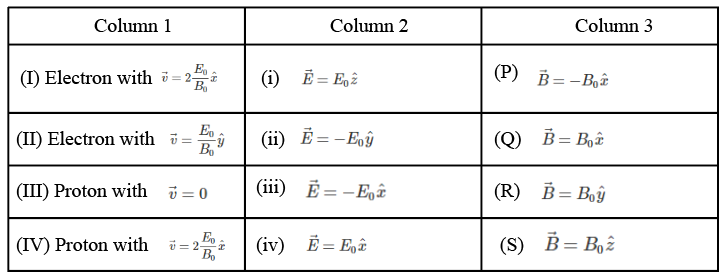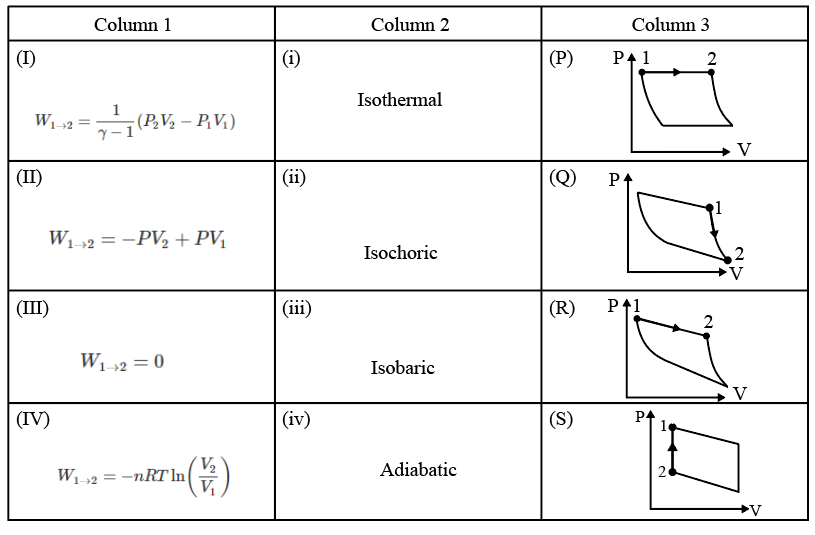Instructions

For the following questions answer them individually

Question 11

Backspace
789
456
123
0.-
Clear All
Question 12

# $$^{131}I$$ is an isotope of Iodine that $$\beta$$ decays to an isotope of Xenon with a half-life of 8 days. A small amount of a serum labelled with $$^{131}I$$ is injected into the blood of a person. The activity of the amount of $$^{131}I$$ injected was $$2.4 \times 10^5$$ Becquerel (Bq). It is known that theinjected serum will get distributed uniformly in the blood stream in less than half an hour. After 11.5 hours, 2.5 ml of blood is drawn from the person’s body, and gives an activity of 115 Bq. The total volume of blood in the person’s body, in liters is approximately (you may use $$e^x \approx 1 + x$$ for $$\mid x \mid \ll 1$$ and $$\ln 2 \approx 0.7$$).

Backspace
789
456
123
0.-
Clear All
Instructions

Answer the Questions by appropriately matching the information given in the three columns of the following table.

A charged particle (electron or proton) is introduced at the origin $$(x = 0, y = 0, z = 0)$$ with a given initial velocity $$\overrightarrow{v}$$. A uniform electric field $$\overrightarrow{E}$$ and a uniform magnetic field $$\overrightarrow{B}$$ exist everywhere. The velocity $$\overrightarrow{v}$$, electric field $$\overrightarrow{E}$$ and magnetic field $$\overrightarrow{B}$$ are given in columns 1, 2 and 3 respectively. The quantities $$E_0, B_0$$ are positive in magnitude.Question 13

Question 14

Question 15

# In which case would the particle move in a straight line along the negative direction of y-axis (i.e., move along $$-\hat{y}$$)?

Instructions

Answer the Questions by appropriately matching the information given in the three columns of the following table.

An ideal gas is undergoing a cyclic thermodynamic process in different ways as shown in the corresponding P - V diagrams in column 3 of the table. Consider only the path from state 1 to state 2. W denotes the corresponding work done on the system. The equations and plots in the table have standard notations as used in thermodynamic processes. Here $$\gamma$$ is the ratio of heat capacities at constant pressure and constant volume. The number of moles in the gas is n.Question 16

Question 17

Question 18

# Which one of the following options correctly represents a thermodynamic process that is used as a correction in the determination of the speed of sound in an ideal gas?

Instructions

For the following questions answer them individually

Question 19

Question 20

OR